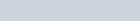# Non-Parametric Tests in Statistics

In statistics, Non parametric tests test which does not make any assumption as to the form of distribution in the population from which the sample is drawn i.e. to say that the functional form of the distributions is not known. The test is called non parametric tests or distribution free test.

### Assumptions of Non parametric test

Non-parametric tests follow some assumptions. They are,

• Sample observations are independent
• The variable under study is continuous.
• The pdf is continuous.
• Lower order moment exists.

Most used non-parametric Tests

There are some test which are generally used widely.

There are some advantages of non parametric test,

• Non parametric methods are readily comprehensive very simple and easy to apply and do not require complicated sample theory.
• No assumption is made when the sample is drawn from population.
• Non- parametric tests are available in deal with the data which are given in rank.

• It can be used only if the measurements are nominal and ordinal even in that case if a parametric test exists it is more powerful than non-parametric test.
• Non Parametric tests are designed to test statistical hypothesis only and not for estimated the parameter.
• A large number of different type of table is required.

### The steps for testing procedure of Non-parametric test

Step 1 : Hypothesis test

State the null hypothesis  (Ho) and it’s alternative hypothesis (H1)

Step 2 : Statistical test

Among several test which might be given research design, choose that test the which most closely approximates the conditional research in terms of the assumptions the test based.

Step-3 : Significance level

Specify a level of significance (α)  and size (n )

Step-4: Sampling distribution

finding the sampling distribution statistic test under the assumption that Ho.

Step 5 : Critical Region

In the basis of step 2,3 and 4 above the region of rejection for the statistic form

Step 6 : Decision

If the value of the test statistic is on of rejection, the decision is to reject wise accept.

### Difference between Parametric and Non-parametric test

Homogeneity of variance for parametric test is,Homogeneity of variance for non-parametric test is,